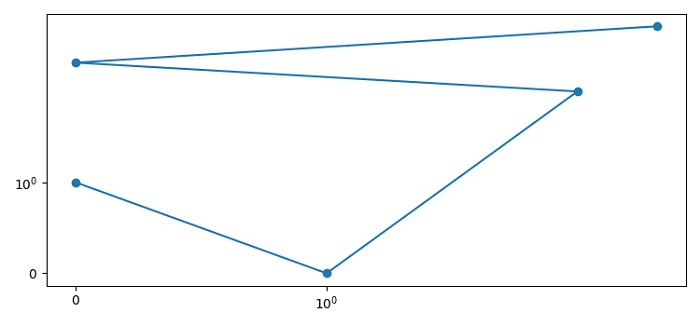# Logscale plots with zero values in Matplotlib

To logscale plots with zero values in matplotlib, we can use xscale() and yscale() methods with "symlog" class by name.

## Steps

• Set the figure size and adjust the padding between and around the subplots.

• Plot two lists containing zero values using plot() method.

• Use yscale() method with "symlog" class by name.

• Use xscale() method with "symlog" class by name.

• To display the figure, use show() method.

## Example

from matplotlib import pyplot as plt
plt.rcParams["figure.figsize"] = [7.50, 3.50]
plt.rcParams["figure.autolayout"] = True
plt.plot([0, 1, 2, 0, 3], [1, 0, 2, 3, 5], marker='o', linestyle='-')
plt.yscale('symlog')
plt.xscale('symlog')
plt.show()

## Output